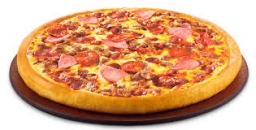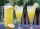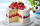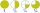# Mixed divided with frac

There are 2 2/3 pizzas. How many people are sharing when each has 2/3 of pizza?

n =  4

### Step-by-step explanation:Did you find an error or inaccuracy? Feel free to write us. Thank you!Tips to related online calculators
Need help to calculate sum, simplify or multiply fractions? Try our fraction calculator.

## Related math problems and questions:

• Barbara 2Barbara get 6 pizzas to divide equally among 4 people. How much of a pizza can each person have?
• PizzasBilly ate 1 1/4 pizzas and John ate 1 2/3 pizzas. How much more pizza did John eat than Billy?
• Two pizzasJacobs mom bought two whole pizzas. He ate 2/10 of the pizza and his dad ate 1 1/5. How much is left.
• Pizza 5You have 2/4 of a pizza, and you want to share it equally between 2 people. How much pizza does each person get?
• EvaluateThe division of numbers $a and$b increase by-product of the numbers $c and$d
• Equivalent expressionsA coach took his team out for pizza after their last game. There were 14 players, so they had to sit in smaller groups at different tables. Six players sat at one table and got 4 small pizzas to share equally. The other players sat at the different table
• Birthday partyJay brought 2 pizzas for his birthday party. At the birthday party, they eye 1 4/7 pizzas . What’s the fraction of pizzas they have left?Six friends are sharing 8 cups of lemonade. If they share the lemonade equally, how many cups will each friend get?
• Pizza fractionsAnn ate a third of a pizza and then another quater. Total part of pizza eaten by Ann and how much pizza is left?
• Pizza palaceJosh is at Enzo's pizza palace. He can sit at a table with 5 of his friends or at a different table with seven of his friends. The same size pizza is shared equally among the people at each table. At which table should Josh sit to get more pizza? (write a
• Numbers divisionWith what number should be divided mixed number 2 3/4 to get 11/12?
• A pizza 2A pizza is cut into slices that are each 1/6 of the whole. John is going to eat 1/2 of the whole pizza. How many slices will John eat?
• Double division0.25 divided by 1/2 divided by 14
• Evaluate mixed expressionsWhich of the following is equal to 4 and 2 over 3 divided by 3 and 1 over 2? A. 4 and 2 over 3 times 3 and 2 over 1 B. 14 over 3 times 2 over 7 C. 14 over 3 times 7 over 2 D. 42 over 3 times 2 over 31
• Four peopleFour people want waffles for breakfast. There are 6 waffles left. How can 6 waffles be shared equally among 4 people? How much does each person get? Draw a picture and write a division expression to model the problem.
• JerryJerry has 3/4 of a pizza. He needs to share it with 6 friends. What fraction of the pizza will each friend get? Only write the fraction
• Two dividedTwo divided by nine-tenths.